# Abstract analytic function

(diff) ← Older revision | Latest revision (diff) | Newer revision → (diff)

analytic mapping of Banach spaces

A functionacting from some domainof a Banach spaceinto a Banach space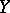that is differentiable according to Fréchet everywhere in, i.e. is such that for any point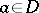there exists a bounded linear operatorfromintofor which the following relation is true: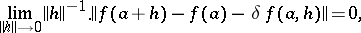where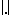denotes the norm onor on;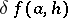is called the Fréchet differential ofat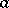.

Another approach to the notion of an abstract analytic function is based on differentiability according to Gâteaux. A functionfromintois weakly analytic in, or differentiable according to Gâteaux in, if for each continuous linear functionalonand each element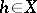the complex function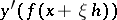is a holomorphic function of the complex variablein the disc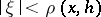, where. Any abstract analytic function in a domainis continuous and weakly analytic in. The converse proposition is also true, and the continuity condition can be replaced by local boundedness or by continuity according to Baire.

The term "abstract analytic function" is sometimes employed in a narrower sense, when it means a function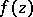of a complex variablewith values in a Banach space or even in a locally convex linear topological space. In such a case any weakly analytic functionin a domainof the complex plane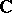is an abstract analytic function. One can also say that a functionis an abstract analytic function in a domain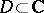if and only ifis continuous inand if for any simple closed rectifiable contour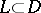the integral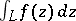vanishes. For an abstract analytic functionof a complex variablethe Cauchy formula (cf. Cauchy integral) is valid.

Let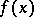be a weakly analytic function in a domainof a Banach space. Then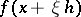, as a function of the complex variable, has derivatives of all orders in the domain,, these derivatives being abstract analytic functions frominto. If the setbelongs to, thenwhere the series converges in norm, and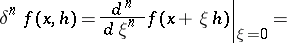A functionfromintois called a polynomial with respect to the variableof degree at mostif, for alland for all complex, one has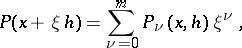where the functions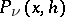are independent of. The degree of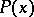is exactlyif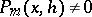. A power series is a series of the formwhere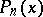are homogeneous polynomials of degreeso that,, for all complex. An arbitrary weakly convergent power series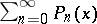in a domainconverges in norm towards some weakly analytic functionin, and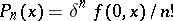,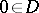. A functionis an abstract analytic function if and only if it can be developed in a power series in a neighbourhood of all pointswhere all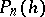are continuous in.

Many fundamental results in the classical theory of analytic functions — such as the maximum-modulus principle, the uniqueness theorems, the Vitali theorem, the Liouville theorem, etc. — are applicable to abstract analytic functions if suitable changes are introduced. The set of all analytic functions in a domainforms a linear space.

The notion of an abstract analytic function can be generalized to wider classes of spacesand, such as locally convex topological spaces, Banach spaces over an arbitrary complete valuation field, etc.

How to Cite This Entry:
Abstract analytic function. Encyclopedia of Mathematics. URL: http://encyclopediaofmath.org/index.php?title=Abstract_analytic_function&oldid=17260
This article was adapted from an original article by A.A. DanilevichE.D. Solomentsev (originator), which appeared in Encyclopedia of Mathematics - ISBN 1402006098. See original article